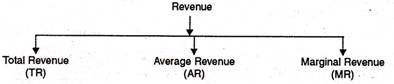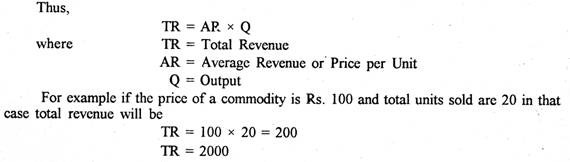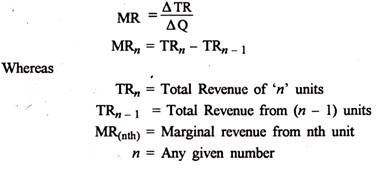# Revenue Analysis

The revenue of a firm jointly with its costs ascertains profits. Now let us discuss the concepts of revenue. The term revenue denotes to the receipts obtained by a firm from the scale of definite quantities of a commodity at various prices. The revenue concept relates to total revenue, average revenue and marginal revenue.1. Total Revenue

The income earned by a seller or producer after selling the output is called the total revenue. In fact, total revenue is the multiple of price and output. The behavior of total revenue depends on the market where the firm produces or sells.

“Total revenue is the sum of all sales, receipts or income of a firm.” Dooley

Total revenue may be defined as the “product of planned sales (output) and expected selling price.” Clower and Due

“Total revenue at any output is equal to price per unit multiplied by quantity sold.” Stonier and Hague1. Average Revenue

Average revenue refers to the revenue obtained by the seller by selling the per unit commodity. It is obtained by dividing the total revenue by total output.

“The average revenue curve shows that the price of the firm’s product is the same at each level of output.” Stonier and Hague1. Marginal Revenue

Marginal revenue is the net revenue obtained by selling an additional unit of the commodity. “Marginal revenue is the change in total revenue which results from the sale of one more or one less unit of output.” Ferguson. Thus, marginal revenue is the addition made to the total revenue by selling one more unit of the good. In algebraic terms, marginal revenue is the net addition to the total revenue by selling n units of a commodity instead of n – 1.1. Koutsoyiannis, “The marginal revenue is the change in total revenue resulting from selling an additional unit of the commodity.”

If total revenue from (n) units is 110 and from (n – 1) units is 100.

In that case

MRnth = TRn – TRn _ 1 = 100 – 100

MRnth = 10

MR in mathematical terms is the ratio of change in total revenue to change in output

MR = ∆TR/∆q or dR/dq = MR

Importance of Revenue Costs

The AR and MR curves form significant tool for economic analysis.

(i) Profit Determinants: The A curve is the price line for the producer in all market situations. By relating the AR curve to the AC curve of a firm, it can ascertain whether it is earning supernormal or normal profits or incurring losses. If the AR curve is tangent to the AC curve at the point of equilibrium, the firm earns normal profits. If the AR curve is above AC curve, it makes super normal profits. In case, AR curve is below the AC curve at the equilibrium point, the firm incurs losses.

(ii) Determination of Full capacity: It can also be known from their relationship whether the firm is producing at is full capacity or under capacity. If the AR curve is tangent to the AC curve at its minimum point, under perfect rivalry, the firm produces its full capacity. Where it is not so, under monopolistic competition, the firm posses idle capacity.

(iii) Equilibrium Determination: The MR curve when intersected by the MC curve determines the equilibrium position of the firm under all market conditions. Their point of intersection in fact determines price, output, and profit and loss of a firm.

(iv) Factor Pricing Determination: The use of the average marginal revenue helps in determining factor prices. In factor pricing they are inverted U shaped and the average and marginal revenue curves become the average revenue productivity and marginal revenue productivity curves ARP and MRP, also they are useful device in describing the equilibrium of the firm under different market conditions.#### intactone

View all posts by intactone →
error: Content is protected !!